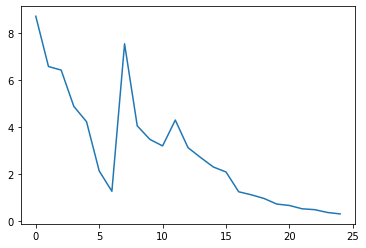Two weeks ago, I presented GPopt: a Python package for Bayesian optimization. In particular, I’ve presented a way to stop the optimizer and resume it later by adding more iterations.

This week, I present a way to save and resume, that makes the optimizer’s data persistent. Behind this saving feature, are hidden Python shelves which are – sort of – hash tables on disk.

We start by installing packages necessary for the demo.

!pip install GPopt
!pip install matplotlib==3.1.3


Import packages.

import GPopt as gp
import numpy as np
import matplotlib.pyplot as plt


Objective function to be minimized.

# branin
def branin(x):
x1 = x
x2 = x
term1 = (x2 - (5.1*x1**2)/(4*np.pi**2) + (5*x1)/np.pi - 6)**2
term2 = 10*(1-1/(8*np.pi))*np.cos(x1)
return (term1 + term2 + 10)


Start the optimizer, and save on disk after 25 iterations.

print("Saving after 25 iterations")
gp_opt3 = gp.GPOpt(objective_func=branin,
lower_bound = np.array([-5, 0]),
upper_bound = np.array([10, 15]),
n_init=10, n_iter=25,
save = "./save") # will create save.db in the current directory
gp_opt3.optimize(verbose=1)
print("current number of iterations:")
print(gp_opt3.n_iter)
gp_opt3.close_shelve()
print("\n")
print("current minimum:")
print(gp_opt3.x_min)
print(gp_opt3.y_min)
plt.plot(gp_opt3.max_ei)

current number of iterations:
25

current minimum:
[3.17337036 2.07962036]
0.4318831996378023


#On this figure, we observe that there’s still room for advancement in the convergence of expected improvement (EI). We can add more iterations to the procedure by loading the saved object.

print("---------- \n")
lower_bound = np.array([-5, 0]),
upper_bound = np.array([10, 15]))
print("current number of iterations:")
gp_optload.optimize(verbose=2, n_more_iter=190, abs_tol=1e-4) # early stopping based on expected improvement
print("current number of iterations:")
print("\n")
print("current minimum:")

current number of iterations: